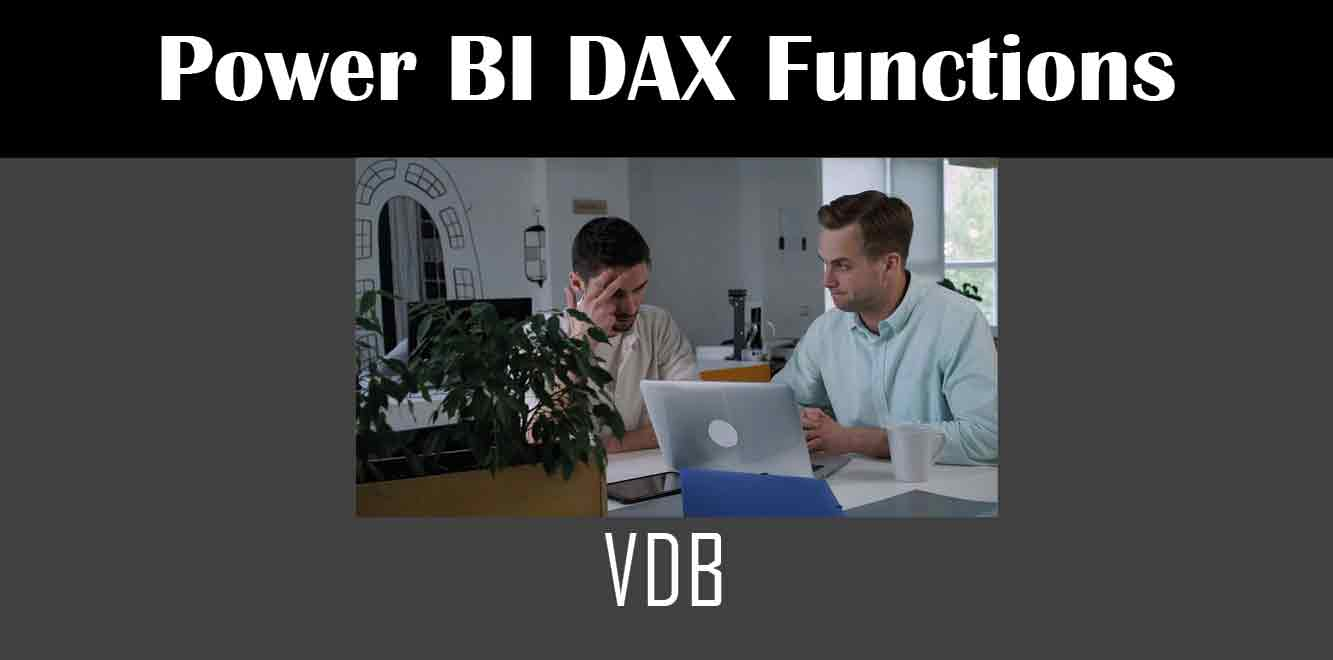# VDB

## Y## What is the VDB Function?

The VDB function is a financial function in DAX that stands for Variable Declining Balance. It is used to calculate the depreciation of an asset over a period of time. The VDB function returns the depreciation amount of an asset for a specific period, using the double-declining balance method or another user-defined method. The syntax for the VDB function is as follows:

VDB (cost, salvage, life, start_period, end_period, [factor], [no_switch])

Where:

– cost: the initial cost of the asset

– salvage: the salvage value of the asset

– life: the expected life of the asset

– start_period: the start period of the depreciation

– end_period: the end period of the depreciation

– factor: the factor used to calculate depreciation (optional)

– no_switch: a logical value that determines whether to switch to straight-line calculation when depreciation comes to salvage value (optional)

## How to Use the VDB Function in Power BI?

To use the VDB function in Power BI, follow these steps:

1. Open Power BI Desktop

2. Load the data into Power BI using the Get Data button

3. Create a new measure by going to the Modeling tab and clicking on New Measure

4. Enter the VDB formula in the formula bar

For example, to calculate the depreciation of an asset with an initial cost of \$10,000, a salvage value of \$1,000, an expected life of 5 years, and a start period of 1, use the following formula:

Depreciation = VDB(10000,1000,5,1,5,2,TRUE)

``` In this example, the factor used to calculate depreciation is 2, and the no_switch parameter is set to TRUE. Tips for Using the VDB Function in Power BI Here are some tips for using the VDB function in Power BI: 1. Make sure that the start period and end period are in the same units (e.g., years, months, days). 2. Use a factor that is appropriate for your asset. A factor of 2 is commonly used for the double-declining balance method, but you can use a custom factor if needed. 3. Set the no_switch parameter to TRUE if you want Power BI to switch to straight-line calculation when depreciation comes to the salvage value. 4. If you have multiple assets with different depreciation rates, create a separate measure for each asset. In this article, we have learned about the VDB function in Power BI and how it can be used to calculate the depreciation of an asset. The VDB function is a powerful tool for financial analysis and can save a lot of time and effort in calculating asset depreciation. By following the tips outlined in this article, you can use the VDB function in Power BI to create accurate and efficient depreciation calculations. Power BI DAX Training Courses by G Com Solutions (0800 998 9248)Power BI DAX Intensive Training Course £1,050.00 – £26,550.00 Select optionsContinue Loading DonePower BI DAX Introduction £395.00 – £9,750.00 Select optionsContinue Loading DonePower BI DAX Intermediate £395.00 – £9,750.00 Select optionsContinue Loading DonePower BI DAX Advanced £395.00 – £9,750.00 Select optionsContinue Loading Done Upcoming Courses 6-8 Jun 23 (London or Online)8-10 Aug 23 (London or Online)24-26 Oct 23 (London or Online) Contact Us Subject Your Name (required) Company/Organisation Email (required) Telephone Training Course(s) Power BI Intensive TrainingPower BI introduction Power BI IntermediatePower BI AdvancedDAXPower Query MPower BI CertificationPower BI AdministrationPower PlatformPower AutomatePower AppsOTHER Your Message Upload Example Document(s) (Zip multiple files) ```
``` ```
``` ```
``` ```
``` ```
``` ```